Anzeige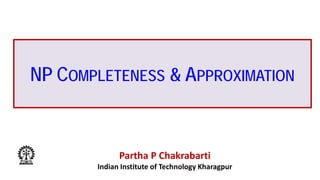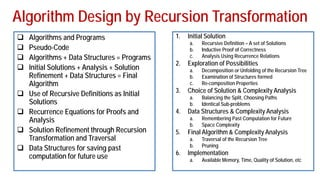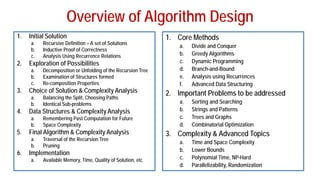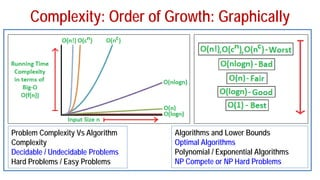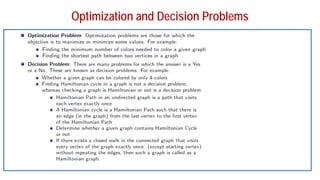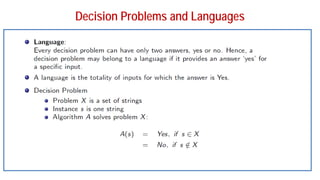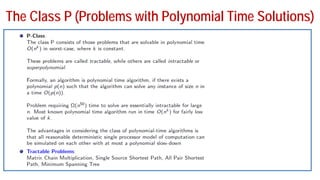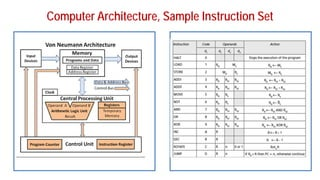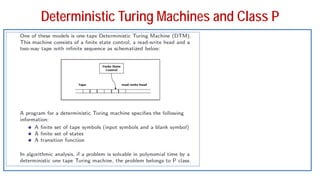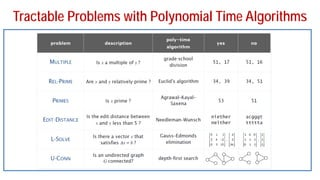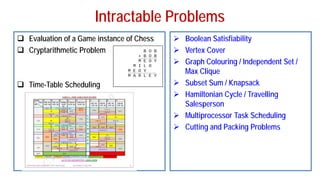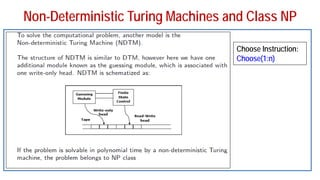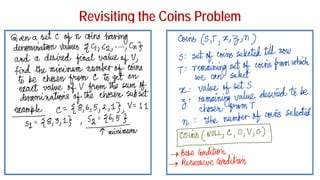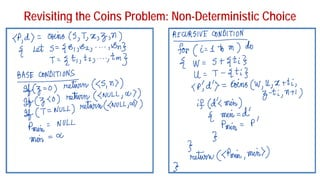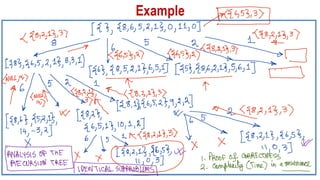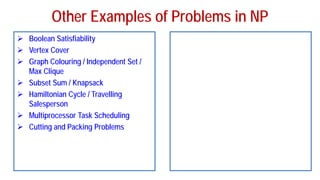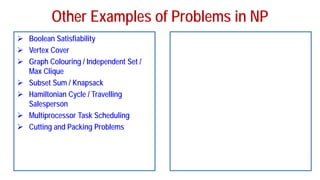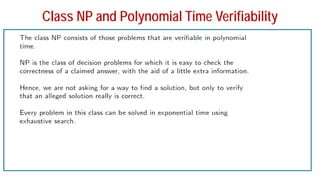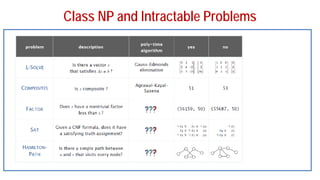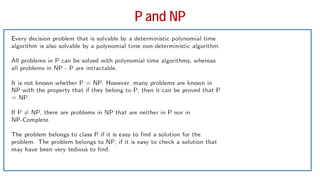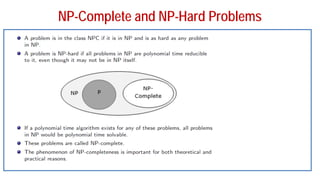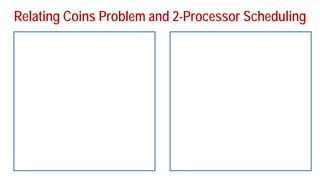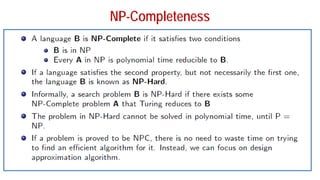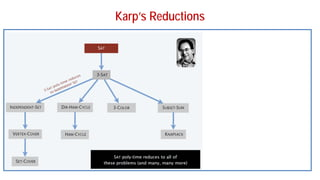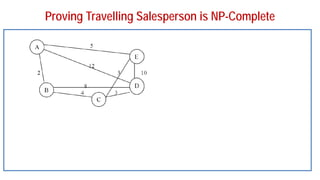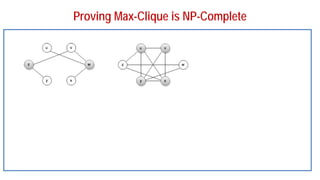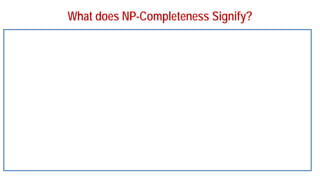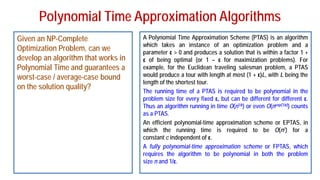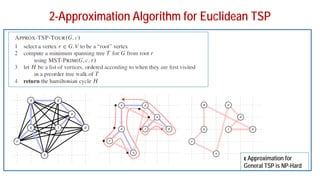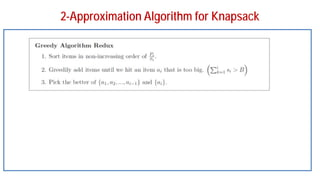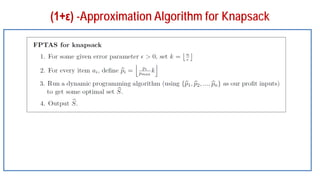1 von 34
Anzeige

### FADML 09 PPC NP Completeness (1).pdf

1. NP COMPLETENESS & APPROXIMATION Partha P Chakrabarti Indian Institute of Technology Kharagpur
2. Algorithm Design by Recursion Transformation 1. Initial Solution a. Recursive Definition – A set of Solutions b. Inductive Proof of Correctness c. Analysis Using Recurrence Relations 2. Exploration of Possibilities a. Decomposition or Unfolding of the Recursion Tree b. Examination of Structures formed c. Re-composition Properties 3. Choice of Solution & Complexity Analysis a. Balancing the Split, Choosing Paths b. Identical Sub-problems 4. Data Structures & Complexity Analysis a. Remembering Past Computation for Future b. Space Complexity 5. Final Algorithm & Complexity Analysis a. Traversal of the Recursion Tree b. Pruning 6. Implementation a. Available Memory, Time, Quality of Solution, etc  Algorithms and Programs  Pseudo-Code  Algorithms + Data Structures = Programs  Initial Solutions + Analysis + Solution Refinement + Data Structures = Final Algorithm  Use of Recursive Definitions as Initial Solutions  Recurrence Equations for Proofs and Analysis  Solution Refinement through Recursion Transformation and Traversal  Data Structures for saving past computation for future use
3. Overview of Algorithm Design 1. Initial Solution a. Recursive Definition – A set of Solutions b. Inductive Proof of Correctness c. Analysis Using Recurrence Relations 2. Exploration of Possibilities a. Decomposition or Unfolding of the Recursion Tree b. Examination of Structures formed c. Re-composition Properties 3. Choice of Solution & Complexity Analysis a. Balancing the Split, Choosing Paths b. Identical Sub-problems 4. Data Structures & Complexity Analysis a. Remembering Past Computation for Future b. Space Complexity 5. Final Algorithm & Complexity Analysis a. Traversal of the Recursion Tree b. Pruning 6. Implementation a. Available Memory, Time, Quality of Solution, etc 1. Core Methods a. Divide and Conquer b. Greedy Algorithms c. Dynamic Programming d. Branch-and-Bound e. Analysis using Recurrences f. Advanced Data Structuring 2. Important Problems to be addressed a. Sorting and Searching b. Strings and Patterns c. Trees and Graphs d. Combinatorial Optimization 3. Complexity & Advanced Topics a. Time and Space Complexity b. Lower Bounds c. Polynomial Time, NP-Hard d. Parallelizability, Randomization
4. Complexity: Order of Growth: Graphically Problem Complexity Vs Algorithm Complexity Decidable / Undecidable Problems Hard Problems / Easy Problems Algorithms and Lower Bounds Optimal Algorithms Polynomial / Exponential Algorithms NP Compete or NP Hard Problems
5. Optimization and Decision Problems
6. Decision Problems and Languages
7. The Class P (Problems with Polynomial Time Solutions)
8. Computer Architecture, Sample Instruction Set
9. Deterministic Turing Machines and Class P
10. Tractable Problems with Polynomial Time Algorithms
11. Intractable Problems  Evaluation of a Game instance of Chess  Cryptarithmetic Problem  Time-Table Scheduling  Boolean Satisfiability  Vertex Cover  Graph Colouring / Independent Set / Max Clique  Subset Sum / Knapsack  Hamiltonian Cycle / Travelling Salesperson  Multiprocessor Task Scheduling  Cutting and Packing Problems
12. Non-Deterministic Turing Machines and Class NP Choose Instruction: Choose(1:n)
13. Revisiting the Coins Problem
14. Revisiting the Coins Problem: Non-Deterministic Choice
15. Other Examples of Problems in NP  Boolean Satisfiability  Vertex Cover  Graph Colouring / Independent Set / Max Clique  Subset Sum / Knapsack  Hamiltonian Cycle / Travelling Salesperson  Multiprocessor Task Scheduling  Cutting and Packing Problems
16. Other Examples of Problems in NP  Boolean Satisfiability  Vertex Cover  Graph Colouring / Independent Set / Max Clique  Subset Sum / Knapsack  Hamiltonian Cycle / Travelling Salesperson  Multiprocessor Task Scheduling  Cutting and Packing Problems
17. Other Examples of Problems in NP  Boolean Satisfiability  Vertex Cover  Graph Colouring / Independent Set / Max Clique  Subset Sum / Knapsack  Hamiltonian Cycle / Travelling Salesperson  Multiprocessor Task Scheduling  Cutting and Packing Problems
18. Class NP and Polynomial Time Verifiability
19. Class NP and Intractable Problems
20. P and NP
21. NP-Complete and NP-Hard Problems
22. Relating Coins Problem and 2-Processor Scheduling
23. NP-Completeness
24. Cook-Levine Theorem (Boolean Satisfiability is NP-Complete)
25. Karp’s Reductions
26. Proving Travelling Salesperson is NP-Complete
27. Proving Max-Clique is NP-Complete
28. What does NP-Completeness Signify?
29. Polynomial Time Approximation Algorithms Given an NP-Complete Optimization Problem, can we develop an algorithm that works in Polynomial Time and guarantees a worst-case / average-case bound on the solution quality? A Polynomial Time Approximation Scheme (PTAS) is an algorithm which takes an instance of an optimization problem and a parameter ε > 0 and produces a solution that is within a factor 1 + ε of being optimal (or 1 – ε for maximization problems). For example, for the Euclidean traveling salesman problem, a PTAS would produce a tour with length at most (1 + ε)L, with L being the length of the shortest tour. The running time of a PTAS is required to be polynomial in the problem size for every fixed ε, but can be different for different ε. Thus an algorithm running in time O(n1/ε) or even O(nexp(1/ε)) counts as a PTAS. An efficient polynomial-time approximation scheme or EPTAS, in which the running time is required to be O(nc) for a constant c independent of ε. A fully polynomial-time approximation scheme or FPTAS, which requires the algorithm to be polynomial in both the problem size n and 1/ε.
30. 2-Approximation Algorithm for Euclidean TSP ε Approximation for General TSP is NP-Hard
31. 2-Approximation Algorithm for Knapsack
32. (1+ε) -Approximation Algorithm for Knapsack
33. Thank you Any Questions?
Anzeige